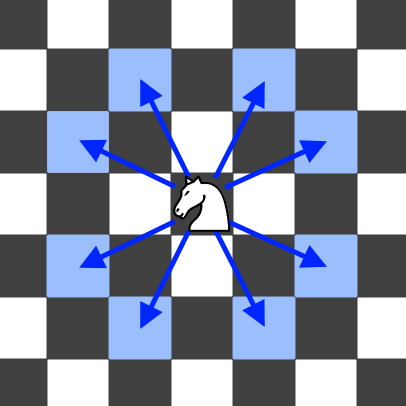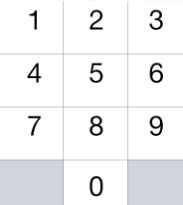Formatted question description: https://leetcode.ca/all/935.html

# 935. Knight Dialer (Medium)

A chess knight can move as indicated in the chess diagram below:.This time, we place our chess knight on any numbered key of a phone pad (indicated above), and the knight makes N-1 hops.  Each hop must be from one key to another numbered key.

Each time it lands on a key (including the initial placement of the knight), it presses the number of that key, pressing N digits total.

How many distinct numbers can you dial in this manner?

Since the answer may be large, output the answer modulo 10^9 + 7.

Example 1:

Input: 1 Output: 10

Example 2:

Input: 2 Output: 20

Example 3:

Input: 3 Output: 46

Note:

• 1 <= N <= 5000

## Solution 1. DP

// OJ: https://leetcode.com/problems/knight-dialer/

// Time: O(N)
// Space: O(1)
class Solution {
vector<vector<int>> next { {4, 6}, {6, 8}, {7, 9}, {4, 8}, {0, 3, 9}, {}, {0, 1, 7}, {2, 6}, {1, 3}, {2, 4} };
public:
int knightDialer(int N) {
vector<vector<int>> dp(2, vector<int>(10, 1));
int mod = 1e9 + 7, ans = 0;
for (int i = 2; i <= N; ++i) {
for (int j = 0; j <= 9; ++j) {
dp[i % 2][j] = 0;
for (int n : next[j]) {
dp[i % 2][j] = (dp[i % 2][j] + dp[(i - 1) % 2][n]) % mod;
}
}
}
for (int i = 0; i <= 9; ++i) ans = (ans + dp[N % 2][i]) % mod;
return ans;
}
};


Java

class Solution {
public int knightDialer(int N) {
final int MODULO = 1000000007;
Map<Integer, int[]> adjacentMap = new HashMap<Integer, int[]>();
adjacentMap.put(0, new int[]{4, 6});
adjacentMap.put(1, new int[]{6, 8});
adjacentMap.put(2, new int[]{7, 9});
adjacentMap.put(3, new int[]{4, 8});
adjacentMap.put(4, new int[]{0, 3, 9});
adjacentMap.put(6, new int[]{0, 1, 7});
adjacentMap.put(7, new int[]{2, 6});
adjacentMap.put(8, new int[]{1, 3});
adjacentMap.put(9, new int[]{2, 4});
int[][] dp = new int[N];
for (int i = 0; i < 10; i++)
dp[i] = 1;
for (int i = 1; i < N; i++) {
for (int j = 0; j < 10; j++) {
dp[i][j] = (dp[i][j] + dp[i - 1][adjacent]) % MODULO;
}
}
int sum = 0;
for (int i = 0; i < 10; i++)
sum = (sum + dp[N - 1][i]) % MODULO;
return sum;
}
}